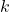## What relevance has theoretical physics to turbulence theory?

The question is of course rhetorical, as I intend to answer it. But I have to pause on the thought that it is also unsatisfactory in some respects. So why ask it then? Well my reply to that is that various turbulence researchers have over the years in effect answered it for me. Their answer would be none at all! In fact, in the case of various anonymous referees, they have often displayed a marked hostility to the idea of theoretical physicists being involved in turbulence research. But the reason why I find it unsatisfactory is that it seems to assume that turbulence theory is not part of theoretical physics, whereas I think it is; or, rather, it should be. So let’s begin by examining that question.

As is well known, the fundamental problem of turbulence is the statistical closure problem that is posed by the hierarchy of moments of the velocity field. Well, molecular physics has the same problem when the molecules interact with each other. This takes the form of the BBGKY hierarchy, although this is expressed in terms of the reduced probability distribution functions. If we consider the simpler problem, where molecules are non-interacting hard spheres, then we have classical statistical physics. In these circumstances we can obtain the energy of the system simply by adding up all the individual energies. The partition function of the system then factorizes, and we can obtain the system free energy quite trivially. However, if the individual molecules are coupled together by an interaction potential, then this factorization is no longer possible as each molecule is coupled to every other molecule in the system. So it is for turbulence, if we work in the Fourier wavenumber representation, the modes of the velocity field are coupled together by the nonlinear term in the velocity field, thus posing an example of what in physics is called the many-body problem.

One could go on with other examples in microscopic physics, for example the theory of magnetism which involves the coupling together of all spins on lattice sites, but it really boils down to the fact that the bedrock problem of theoretical physics is that of strong-coupling. And turbulence formulated in-space comes into that category. The only difference is, that turbulence is mainly studied by engineers and applied scientists, while theorists mostly prefer to study what they see as more fundamental problems, even if these studies become ever more arid for lack of genuine inspiration or creativity. But as a matter of taxonomy, not opinion, turbulence should belong to physics as an example of the many-body problem.

Now let’s turn to our actual question. We can begin by noting that we are talking about insoluble problems. That is, there is no general method of obtaining an exact solution. We have to consider approximate methods. First, there is perturbation theory, which relies on (and is limited by) the ability to perform Gaussian functional integrals. Secondly, there is self-consistent field theory. Both of these rely, either directly or indirectly, on the concept of renormalization. In molecular physics, this involves adding some of the interaction energy to the bare particle, in order to create a dressed particle, also known as a quasi-particle. Such quasi-particles do not interact with each other and so the partition function can be evaluated by factorization, just as in the ideal-gas case. In the case of turbulence, it is probably quite widely recognized nowadays that an effective viscosity may be interpreted as a renormalization of the fluid kinematic viscosity. However, it should be borne in mind that the stirring forces and the interaction strength may also require renormalization.

There is no inherent reason why the subject of statistical turbulence theory should be mysterious and I intend to post short discussions of various aspects. Not so much maths, as `good versus bad’ or `justified versus unjustified’; plus tips on how to use some common sense reasoning to cut through the intimidatingly complicated mathematics and (in some cases self-important pomposity) of some theories which are not really new turbulence theories but merely text-book material from quantum field theory in which variables have been relabelled, but the essential difficulties of extending to turbulence have not been tackled.